# Introducing exdex: Estimation of the Extremal Index

#### 2022-04-15

The extremal value index $$\theta$$ is a measure of the extent of clustering in the extremes of a stationary process, representing the reciprocal of the mean cluster size.

The main purpose of the exdex package is to implement the semiparametric maxima estimators developed in Northrop (2015) and Berghaus and Bücher (2018). A feature of these works is the use of sliding block maxima, that is, the use of all blocks of consecutive values, rather than maxima over disjoint block of values. This improves efficiency of estimation, albeit at the cost of complicating theoretical work. Also provided are functions to estimate $$\theta$$ using two threshold-based methods: the $$K$$-gaps estimator (Süveges and Davison (2010)) and the iterated weighted least squares estimator (Süveges (2007)).

## Semiparametric maxima estimators

These estimators are based on the approximate relation $$G(u_b) = F(u_b)^{b\theta}$$ between the marginal distribution function $$F$$ of the process and the distribution function $$G$$ of the block maximum $$M$$ of $$b$$ consecutive variables, which applies provided that $$b$$ and $$u_b$$ are large. If $$G = F^{b\theta}$$ then $$Y = -b\log F(M)$$ has an exponential($$\theta$$) distribution and $$Z = b(1 - F(M))$$ is a multiple $$b$$ of a beta($$1, b\theta$$) distribution.

Let $$(M_1, \ldots, M_k)$$ be a sample of block maxima of $$b$$ consecutive values. The marginal distribution function $$F$$ is not known so we estimate it using the empirical distribution function $$\hat{F}$$ set $\quad Y_i = -b \log \hat{F}(M_i), \quad Z_i = b(1 - \hat{F}(M_i)), \quad i = 1, \ldots, k$ and define the estimators $\hat{\theta}_N = \left( \frac{1}{k} \sum_{i=1}^k Y_i \right)^{-1} \quad \hat{\theta}_{BB} = \left( \frac{1}{k} \sum_{i=1}^k Z_i \right)^{-1}.$ These two estimators are equivalent asymptotically. The former is the estimator proposed in Northrop (2015). Berghaus and Bücher (2018) derived asymptotic properties of the latter, because it is more amenable to mathematical analysis.

The function spm provides three estimators, estimated using both sliding and disjoint block maxima and four types of bias-adjustment. The estimators are $$\hat{\theta}_N, \hat{\theta}_{BB}$$ and $$\hat{\theta}_{BB}-1/b$$. The latter is motivated by the observation that E$$(Z) = (\theta + 1/b)^{-1} < \theta^{-1}$$ and therefore the subtraction of $$1 / b$$ provides further bias-adjustment. In fact empirical results indicate that $$\hat{\theta}_N$$ and $$\hat{\theta}_{BB} - 1/b$$ produce similar estimates.

### Newlyn sea surges

We provide an illustration of the spm function using the newlyn data, a time series of 2894 maximum seas surges measured at Newlyn, Cornwall, UK over the period 1971-1976. We use a block size $$b = 20$$. We explain in Block size selection how this value was chosen.

library(exdex)
theta <- spm(newlyn, 20)
# Estimates: BB2018b is BB2018 - 1/b
theta
#>
#> Call:
#> spm(data = newlyn, b = 20)
#>
#> Estimates of the extremal index theta:
#>           N2015   BB2018  BB2018b
#> sliding   0.2392  0.3078  0.2578
#> disjoint  0.2350  0.3042  0.2542
summary(theta)
#>
#> Call:
#> spm(data = newlyn, b = 20)
#>
#>                   Estimate Std. Error Bias adj.
#> N2015, sliding      0.2392    0.01990  0.003317
#> BB2018, sliding     0.3078    0.01642  0.003026
#> BB2018b, sliding    0.2578    0.01642  0.053030
#> N2015, disjoint     0.2350    0.02222  0.003726
#> BB2018, disjoint    0.3042    0.02101  0.003571
#> BB2018b, disjoint   0.2542    0.02101  0.053570

There is a confint method for calculating confidence intervals using objects returned from spm. The likelihood-based intervals are based on an adjustment (performed by the chandwich package Northrop and Chandler (2021)) of the naive (pseudo-)loglikelihood so that the curvature of the adjusted loglikelihood agrees with the estimated standard errors.

# Sliding maxima, symmetric intervals
conf <- confint(theta)
# Sliding maxima, likelihood-based intervals
conf <- confint(theta, interval_type = "lik")
plot(conf)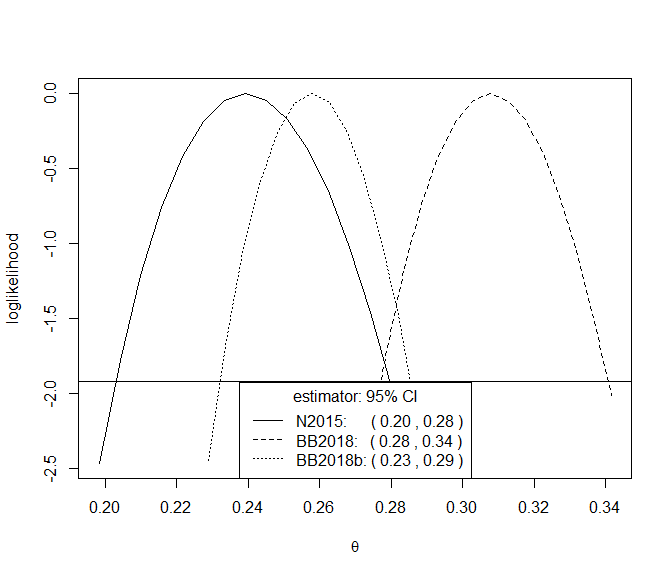For this small block size of $$b = 20$$ there is an appreciable difference between the inferences from the N2015 and BB2018 estimates.

### S&P 500 index

We also consider a dataset (sp500) that is similar to one analysed in Berghaus and Bücher (2018): daily log returns of the S&P 500 index, from 3rd January 1990 to 9th October 2018. We use a block size $$b = 225$$, which is similar to that chosen by Berghaus and Bücher (2018).

theta <- spm(sp500, 225)
summary(theta)
#>
#> Call:
#> spm(data = sp500, b = 225)
#>
#>                   Estimate Std. Error Bias adj.
#> N2015, sliding      0.2454    0.04075   0.01451
#> BB2018, sliding     0.2497    0.04069   0.01452
#> BB2018b, sliding    0.2453    0.04069   0.01897
#> N2015, disjoint     0.2402    0.04150   0.01472
#> BB2018, disjoint    0.2446    0.04152   0.01475
#> BB2018b, disjoint   0.2401    0.04152   0.01920
conf <- confint(theta, interval_type = "lik")
plot(conf)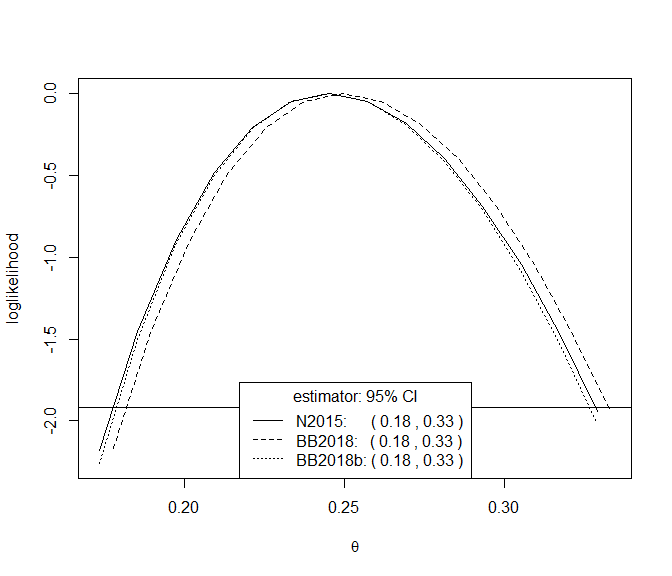For this large block size there is very little difference between the inferences produce by the three estimators.

### Block size selection

An informal way to choose the value of $$b$$ is plots estimates of $$\theta$$ over a range of values of $$b$$ and to select the smallest $$b$$ above which these estimates appear to be constant with respect to $$b$$, taking into account sampling variability. The function choose_b, and its associated plot method, does this, quantifying sampling variability using pointwise confidence intervals. The plot method allows us to select one of 3 estimators and choose whether to use sliding or disjoint block maxima. The default is to plot the estimates using the Northrop (2015) estimator applied to sliding maxima.

For the newlyn data we obtain the following plot.

# Plot like the top left of Northrop (2015)
# We remove the 14 values because 2880 has lots of factors
b_vals <- c(2,3,4,5,6,8,9,10,12,15,16,18,20,24,30,32,36,40,45,48,54,60)
res <- choose_b(newlyn[1:2880], b_vals)
# Some b are too small for the sampling variance of the sliding blocks
# estimator to be estimated
plot(res, ylim = c(0, 1))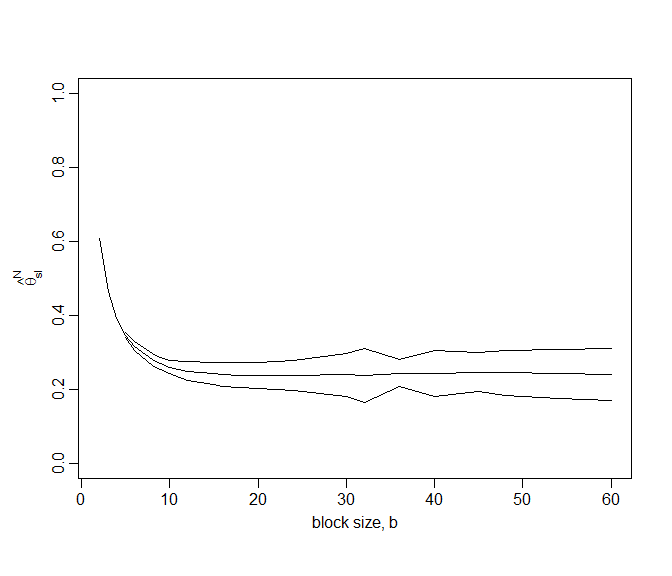This plots suggests that $$b = 20$$ is a reasonable block size to choose. Indeed the point estimates varies smoothly with $$b$$ and varies little for $$b \geq 20$$. The confidence intervals tend to widen as $$b$$ increases, although the method for estimating these intervals is somewhat sensitive to block size owing to its use of disjoint block maxima. See the help file for spm and Berghaus and Bücher (2018) for further information. Note that for very small values of $$b$$ it may not be possible to estimate the confidence intervals, hence the missing intervals in this plot.

For the sp500 data we obtain the following plot.

b_vals <- c(10, seq(from = 25, to = 350, by = 25), 357)
res500 <- choose_b(sp500, b_vals)
plot(res500, ylim = c(0, 1))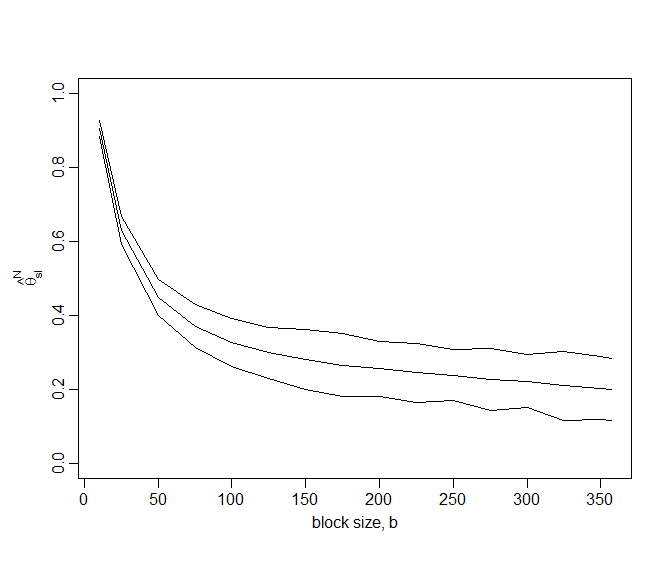The estimates of $$\theta$$ stabilise less quickly than for the newlyn data but $$b = 225$$ seems to be reasonable choice.

## Threshold-based estimators

These estimators are based on the distribution of the times at which observations exceed some high threshold $$u$$. They are based on Ferro and Segers (2003), which uses a limiting ($$u \rightarrow \infty$$) mixture model to represent the distribution of within- and between-cluster interexceedance times. Under the model with probability $$\theta$$ an interexceedance time has an exponential distribution with mean $$1/\theta$$ (a between-cluster time) and otherwise the interexceedance time is, in theory, zero (a within-cluster time).

In practice, zero interexceedance times are impossible and some adjustment is advisable in order to mitigate the effects of the model not fitting well for small interexceedance times. The following estimators take different approaches to solving this problem.

### $$K$$-gaps

Süveges and Davison (2010) to introduce an extra tuning parameter, run parameter $$K$$ that truncates interexceedance times, with the effect that any interexceedance time that is $$\leq K$$ is set to zero. Provided that a suitable value of $$K$$ is chosen $$\theta$$ may be estimated under the mixture model using maximum likelihood estimation. The following code does this using a particular threshold and $$K = 1$$.

u <- quantile(sp500, probs = 0.60)
theta <- kgaps(sp500, u, k = 1)
summary(theta)
#>
#> Call:
#> kgaps(data = sp500, u = u, k = 1)
#>
#>       Estimate Std. Error
#> theta   0.6953   0.007234

Süveges and Davison (2010) propose a diagnostic test, an information matrix text (IMT), to inform the choice of $$(u, K)$$. The test is based on a statistics that compares the Fisher information for $$\theta$$ to the variance of the score statistic, both evaluated at the estimate $$\hat{\theta}$$, because these two quantities are equal for a well-specified regular model. The following code performs this test, using a test of size $$\alpha = 0.05$$, for a range of values of $$u$$ and $$K$$ and produces graphical summary containing a horizontal line to indicator the critical value of the test.

u <- quantile(sp500, probs = seq(0.1, 0.9, by = 0.1))
imt_theta <- choose_uk(sp500, u = u, k = 1:5)
plot(imt_theta, uprob = TRUE, alpha = 0.05)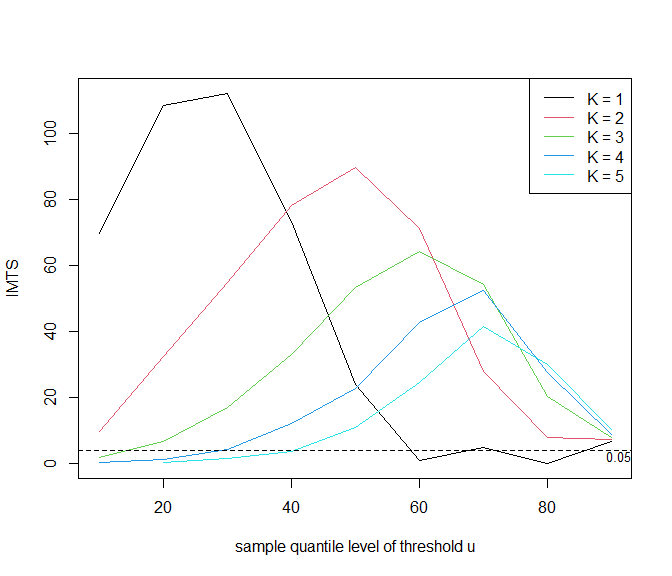Large values of the IMT statistic suggest a poor choice of $$(u, K)$$. Diagnostic plots like this are never clear cut but the fact that for $$K = 1$$ the IMT statistics drops below the critical value at around the sample 60% quantile suggests the combination of $$u$$ and $$K$$ that we used above.

#### Multiple time series and missing values

The cheeseboro dataset contains hourly maximum wind gusts recorded at the Cheeseboro weather station near Thousand Oaks, Southern California, USA during the month of January over the period 2000-2009. Therefore, these data contain 10 time series that we shall consider to be approximately independent of each other. These data also contain several missing values.

summary(cheeseboro)
#>       2000            2001            2002            2003
#>  Min.   : 0.00   Min.   : 3.00   Min.   : 2.00   Min.   : 1.00
#>  1st Qu.: 8.00   1st Qu.:10.00   1st Qu.: 8.00   1st Qu.: 7.00
#>  Median :11.00   Median :15.00   Median :12.00   Median :12.00
#>  Mean   :13.59   Mean   :18.88   Mean   :14.68   Mean   :16.47
#>  3rd Qu.:16.00   3rd Qu.:25.00   3rd Qu.:19.00   3rd Qu.:20.00
#>  Max.   :63.00   Max.   :69.00   Max.   :62.00   Max.   :92.00
#>  NA's   :12      NA's   :9       NA's   :14      NA's   :4
#>       2004            2005            2006            2007           2008
#>  Min.   : 2.00   Min.   : 0.00   Min.   : 2.00   Min.   : 1.0   Min.   : 2.00
#>  1st Qu.: 9.00   1st Qu.: 8.00   1st Qu.: 9.00   1st Qu.: 9.0   1st Qu.:10.00
#>  Median :13.00   Median :13.00   Median :14.00   Median :15.0   Median :15.00
#>  Mean   :15.61   Mean   :16.23   Mean   :18.83   Mean   :18.7   Mean   :17.47
#>  3rd Qu.:20.00   3rd Qu.:22.00   3rd Qu.:25.00   3rd Qu.:27.0   3rd Qu.:23.00
#>  Max.   :53.00   Max.   :51.00   Max.   :71.00   Max.   :60.0   Max.   :53.00
#>                  NA's   :1       NA's   :2
#>       2009
#>  Min.   : 0.00
#>  1st Qu.: 9.00
#>  Median :15.00
#>  Mean   :18.86
#>  3rd Qu.:27.00
#>  Max.   :62.00
#> 

Inside kgaps() these data are divided further into sequences of non-missing values with each sequence stored in a separate column of a matrix. The $$K$$-gaps log-likelihood is constructed as a sum of contributions from columns.

probs <- c(seq(0.5, 0.98, by = 0.025), 0.99)
u <- quantile(cheeseboro, probs = probs, na.rm = TRUE)
imt_theta <- choose_uk(cheeseboro, u, k = 1:10)
plot(imt_theta, uprob = FALSE, lwd = 2)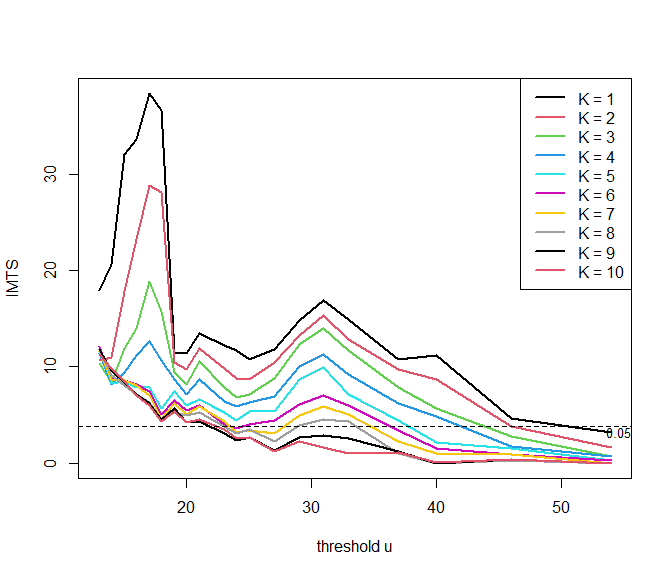For these data the diagnostic graphic for choosing $$(u, K)$$ is perhaps less clear than for the sp500 data. As an example, we use $$u = 45$$ mph and $$K = 3$$ below.

theta <- kgaps(cheeseboro, 45, k = 3)
theta
#>
#> Call:
#> kgaps(data = cheeseboro, u = 45, k = 3)
#>
#> Estimate of the extremal index theta:
#>  theta
#> 0.2405
summary(theta)
#>
#> Call:
#> kgaps(data = cheeseboro, u = 45, k = 3)
#>
#>       Estimate Std. Error
#> theta   0.2405    0.02336

### $$D$$-gaps

From version 1.2.1 of exdex the estimator of $$\theta$$ developed by Holesovsky and Fusek (2020) is available, which involves a censoring parameter $$D$$. This estimator is similar to the $$K$$-gaps estimator, but the treatment of small inter-exceedance times is different. Threshold inter-exceedances times that are not larger than units are left-censored and contribute to a log-likelihood only the information that they are $$\leq D$$. The function dgaps calculates maximum likelihood estimates of $$\theta$$.

theta <- dgaps(cheeseboro, 45, D = 3)
theta
#>
#> Call:
#> dgaps(data = cheeseboro, u = 45, D = 3)
#>
#> Estimate of the extremal index theta:
#>  theta
#> 0.2431
summary(theta)
#>
#> Call:
#> dgaps(data = cheeseboro, u = 45, D = 3)
#>
#>       Estimate Std. Error
#> theta   0.2431    0.02396

### Iterated weighted least squares

Under the limiting mixture model $$\theta$$ is the proportion of interexceedance times that are between clusters. Süveges (2007) uses this to devise and iterated scheme for estimating $$\theta$$. An initial estimate of $$\theta$$ is set. An exponential distribution is fitted to the largest $$100\theta\%$$ of the interexceedance times using weighted least squares estimation. Then the new estimate of $$\theta$$ is used to set the putative exponential sample and the estimation proceeds until the estimate of $$\theta$$ converges.

The current implementation of this estimator in exdex is very limited: only an estimate is produced, with no estimates of uncertainty. The next release of exdex will rectify this.

u <- quantile(newlyn, probs = 0.90)
theta <- iwls(newlyn, u)
theta
#>
#> Call:
#> iwls(data = newlyn, u = u)
#>
#> Convergence (0 means success): 0
#>
#> Estimate of the extremal index theta:
#>   0.2514